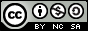- 目录 -
LeetCode 632 最小区间(贪心，堆)

输入:[[4,10,15,24,26], [0,9,12,20], [5,18,22,30]]



1. 给定的列表可能包含重复元素，所以在这里升序表示 >= 。
2. 1 <= k <= 3500
3. -105 <= 元素的值 <= 105
4. 对于使用Java的用户，请注意传入类型已修改为List<List>。重置代码模板后可以看到这项改动。

## 代码

  1 2 3 4 5 6 7 8 9 10 11 12 13 14 15 16 17 18 19 20 21 22 23 24 25 26 27 28 29 30 31 32 33 34 35 36 37 38 39 40  class Solution { public: struct point { int row, pos, v; }; friend bool operator<(point a, point b) { return a.v > b.v; } vector smallestRange(vector> &nums) { int n = nums.size(); priority_queue q; int minn = 0, maxx = INT_MIN; int l = 0, r = INT_MAX; for (int i = 0; i < n; i++) { q.push(point{i, 0, nums[i]}); maxx = max(maxx, nums[i]); } while (!q.empty()) { point p = q.top(); q.pop(); minn = p.v; if (maxx - minn < r - l) { l = minn; r = maxx; } if (p.pos == nums[p.row].size() - 1) break; maxx = max(maxx, nums[p.row][p.pos + 1]); q.push(point{p.row, p.pos + 1, nums[p.row][p.pos + 1]}); } return {l, r}; } };